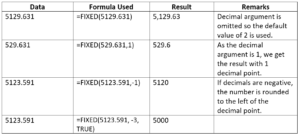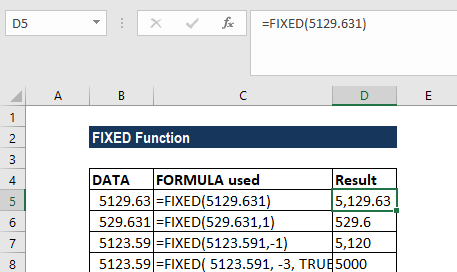# FIXED Function

Returns a text representation of a number rounded to the specified number of decimal places

## What is the FIXED Function?

The FIXED Function is categorized under Excel TEXT functions. It will provide a text representation of a number rounded to the specified number of decimal places.

While doing financial analysis, we often import data from external sources. The FIXED function helps convert numbers into text.

### Formula

=FIXED(number, [decimals], [no_commas])

The FIXED function uses the following arguments:

• Number (required argument) – This is the number that we wish to round off and convert into a text string.
• Decimals (optional argument) – This specifies the number of decimal places to be displayed after the decimal point. So,
• If [decimals] argument is omitted, it takes on the default value of 2;
• If [decimals] argument is negative, the supplied number is rounded up to the left of the decimal point.
• No_commas (optional argument) – This specifies if the returned text should separate number groups (i.e., thousands, millions, etc.) by commas. The possible values are:
• TRUE – Commas are not included in the resulting text;
• FALSE – Commas are included in the resulting text.

If the [no_commas] argument is omitted, it uses the default value of FALSE.

### How to use the FIXED Function in Excel?

As a worksheet function, FIXED can be entered as part of a formula in a cell of a worksheet. To understand the uses of the function, let us consider an example:

#### Example

When we provide the following data using the FIXED function, let us see the result we would get:The results in Excel are shown below:### A few things to remember about the FIXED Function

1. #VALUE! error – Occurs when any of the supplied arguments are non-numeric.
2. Remember that once a number’s been converted using the FIXED function, it is stored in Excel as text. Therefore, it cannot be used in numeric calculations.
3. Numbers in Excel can never include more than 15 significant digits, but decimals can be as large as 127.

Thanks for reading CFI’s guide to important Excel functions! By taking the time to learn and master these functions, you’ll significantly speed up your financial analysis. To learn more, check out these additional CFI resources: